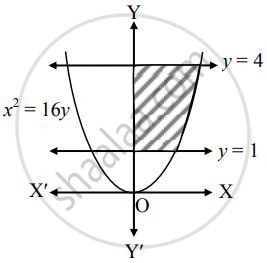# Fill in the blank : Area of the region bounded by x2 = 16y, y = 1, y = 4 and the Y-axis, lying in the first quadrant is _______. - Mathematics and Statistics

Fill in the Blanks

Fill in the blank :

Area of the region bounded by x2 = 16y, y = 1, y = 4 and the Y-axis, lying in the first quadrant is _______.

#### SolutionRequired area = int_1^4x*dy

= int_1^4 4sqrt(y)*dy

= 4[y^(3/2)/(3/2)]_1^4

= (8)/(3)[(4)^(3/2) - (1)^(3/2)]

= (8)/(3)(8 - 1)

= (56)/(3)"sq.units".

Is there an error in this question or solution?
Chapter 7: Applications of Definite Integration - Miscellaneous Exercise 7 [Page 158]

#### APPEARS IN

Balbharati Mathematics and Statistics 1 (Commerce) 12th Standard HSC Maharashtra State Board
Chapter 7 Applications of Definite Integration
Miscellaneous Exercise 7 | Q 2.3 | Page 158
Share